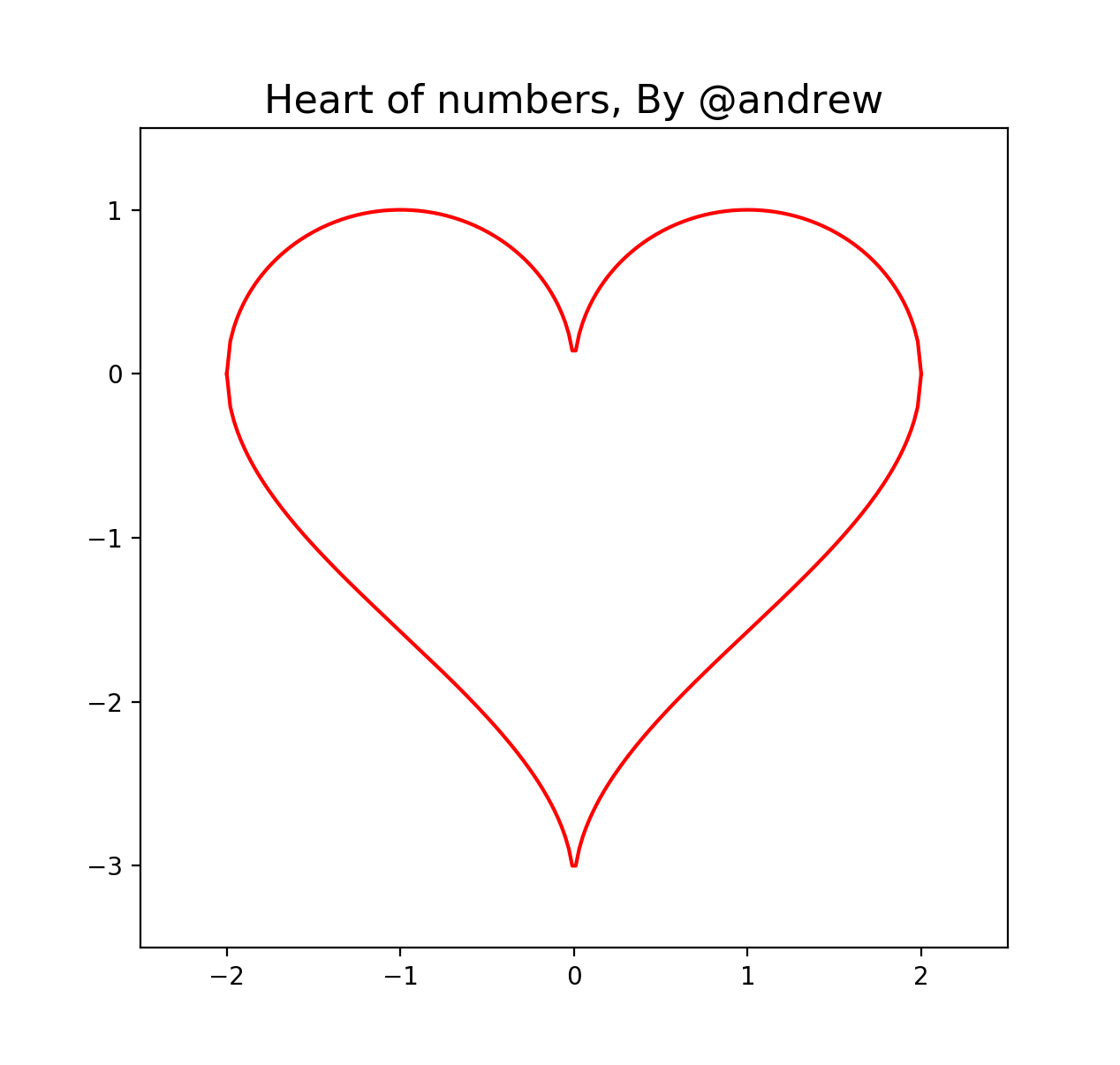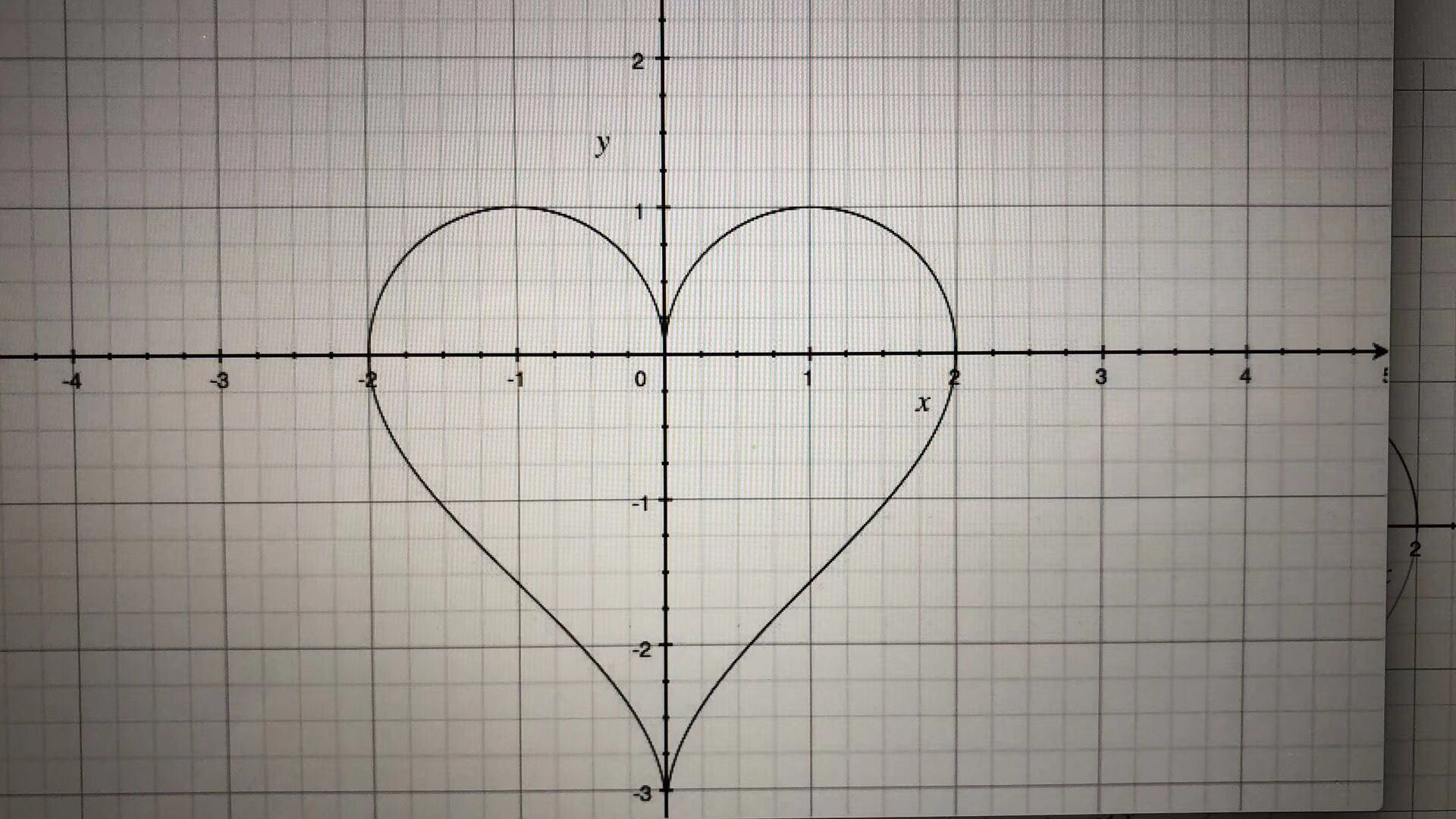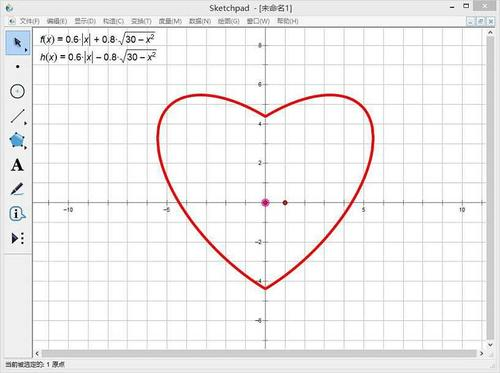# 就算会用python画颗心，可你依然还是只单身狗

Draw a heart in python:) 标题是开玩笑的，千万别认真。$$y = \sqrt{1-(\left| x \right|-1)^2}, arccos(1-\left| x \right|)-\pi$$#!/usr/bin/env python3

import matplotlib.pyplot as plt
import numpy as np

x = np.linspace(-2, 2, 200)
y1 = np.sqrt(1-np.square(np.fabs(x)-1))
y2 = np.arccos(1-np.fabs(x))-np.pi

plt.plot(x, y1, 'r',x,y2,'r')
plt.axis([-2.5, 2.5, -3.5, 1.5])

plt.title('Heart of numbers, By @andrew', fontsize=16)

plt.show()


#### 参考资料

https://www.quora.com/What-is-the-equation-that-gives-you-a-heart-on-the-graph